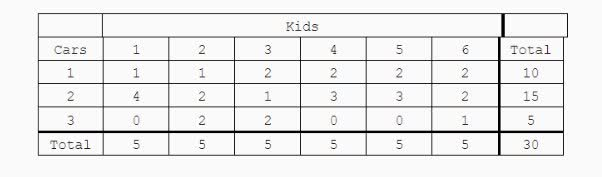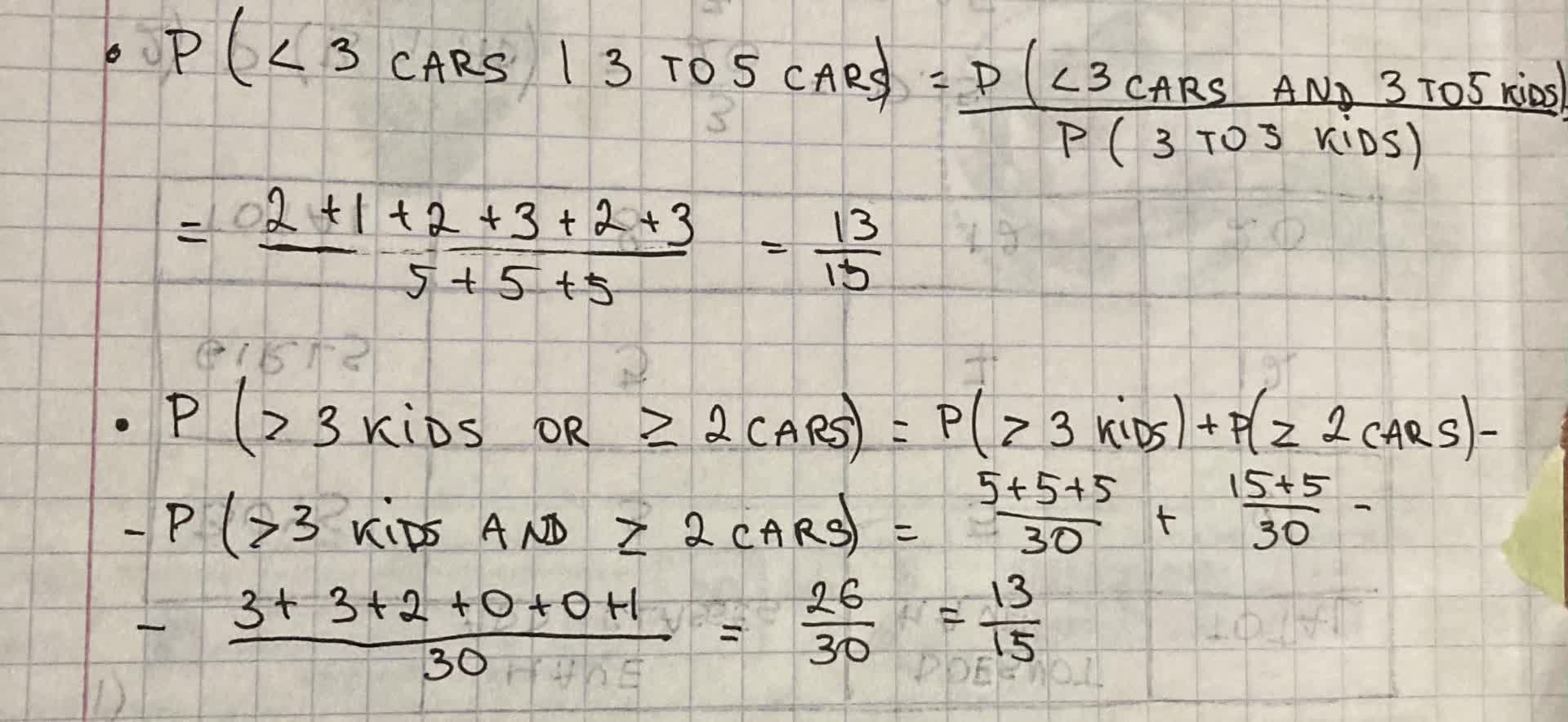# Use the two-way table below and answer the questions. 1) P (< 3 cars | 3 to 5 kids) = ? 2) P ( > 3 kids or >= 2 cars) = ?avissidep 2021-09-02 Answered

Use the two-way table below and answer the questions.
1) P (< 3 cars | 3 to 5 kids) = ?
2) P ( > 3 kids or $\ge$ 2 cars) = ?You can still ask an expert for help

## Want to know more about Two-way tables?

• Questions are typically answered in as fast as 30 minutes

Solve your problem for the price of one coffee

• Math expert for every subject
• Pay only if we can solve ittoroztatG

The answer is given below in the photo